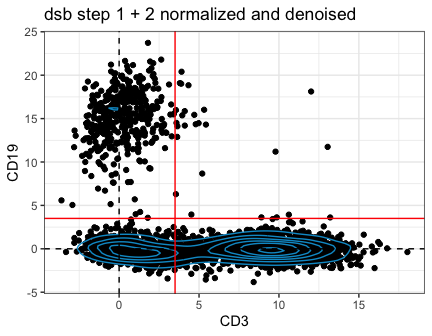The purpose of this document is for users interested in the underlying model works and how the code under the hood of the dsb method is implemented in a step by step manner as outlined in the methods section of the paper. All of the steps outlined below are carried out in a single step with the DSBNormalizeProtein() function.

suppressMessages(library(mclust))
suppressMessages(library(magrittr))
suppressMessages(library(ggplot2))
#> Warning: replacing previous import 'lifecycle::last_warnings' by
#> Warning: replacing previous import 'lifecycle::last_warnings' by
library(dsb) 

cell is a random subset of ~2000 cells from our PBMC data and neg are a subset of background drops.

cell = dsb::cells_citeseq_mtx
neg = dsb::empty_drop_citeseq_mtx

### log transform

Log transform background droplets

# log transformation
dlog = log(cell + 10)
nlog = log(neg + 10)

## dsb step 1 removal of ambient noise

In step I, protein counts in empty droplets are used to estimate the expected ambient background noise for each antibody. Each protein’s counts in cell-containing droplets are thus rescaled using this expected noise measurement.

How do we know this removes the ambient component?
In our experiments outlined in the paper using unstained spike-in control cells added after staining prior to droplet encapsulation, we found that this captures the ambient component of protein specific noise. Background levels in the empty droplets and cells were highly correlated using several definitions of empty / background droplets.

Calculate the mean and standard deviation of the log transformed cells and background droplets:

# calc mean and sd of background drops
sd_nlog = apply(nlog, 1 , sd)
mean_nlog = apply(nlog, 1 , mean)

Standardize ADT levels in the cell-containing droplets based on ambient noise. Subtract background mean $$\mu_{N}$$ and divide by the background standard deviation $$\sigma_{N}$$ of ADTs detected droplets detected in the empty / background droplets. This makes the value the signal above expected background in units of background standard deviations.

$y = (log(x_{i} + P) - µ_{N}) / \sigma_{N}$


norm_adt = apply(dlog, 2, function(x) (x  - mean_nlog) / sd_nlog) 

Below we view the distribution of ambient corrected data compared to a simple log transformation as well as the raw data.

# check structure of denoised data with zero centering of background population
r = 'deepskyblue3'
plist = list(theme_bw(), geom_density_2d(color = r),
geom_vline(xintercept = 0, linetype = 'dashed'),
geom_hline(yintercept = 0, linetype = 'dashed'),
xlab('CD3'), ylab('CD19')
)

p1 = qplot(as.data.frame(t(norm_adt))$CD3_PROT, as.data.frame(t(norm_adt))$CD19_PROT) +
plist +
ggtitle('dsb step 1')

# raw data
p2 = qplot(as.data.frame(t(cell))$CD3_PROT, as.data.frame(t(cell))$CD19_PROT) +
plist +
ggtitle('RAW data')

# log transformed data
p3 = qplot(as.data.frame(t(dlog))$CD3_PROT, as.data.frame(t(dlog))$CD19_PROT) +
plist +
ggtitle('log transformed')

# examine distributions.
cowplot::plot_grid(p1, p2, p3, nrow = 1)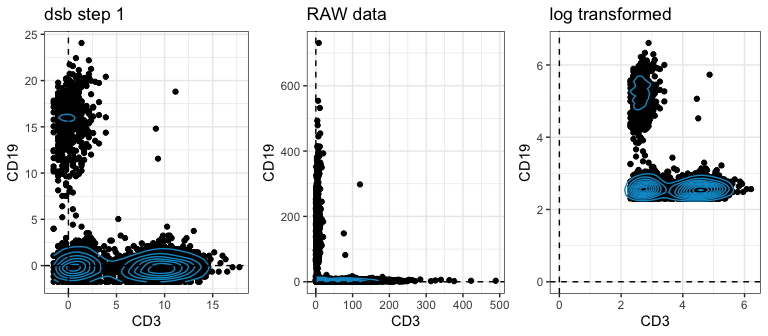## dsb step II Part I fitting single cell models to extract background

In the second step of dsb, we remove technical cell to cell variations.

There are 2 parts to this second step This section describes the first part of the second step: dsb fits a Gaussian mixture with 2 mixing components to model the ADT levels in each cell. This captures the positive-staining proteins and the background, non-staining proteins. Intuitively, we can think of this as in each cell the model is assigning each protein to a positive and negative population, we then take the mean of the proteins in the background. Why do we use a 2 component mixture instead of, say, 3, or 4? Is a 2-component mixture an overly simplistic fit? No–there are several reasons this is the case discussed in detail in the paper. briefly:

1. Based on information criteria, a 2 component model provided the optimal fit in a majority of cells (e.g. more than 85%).
2. Most of the remaining cells have a 3-component mixture as the best fit, however, the only parameter from the fitted model used by dsb, is µ1-the background mean. µ1 was nearly identical with the 2 or 3 component means–this is because often there are many more proteins in the background population than the positive population for a given cell.
3. The observations above generalized to all datasets tested, including those with over 200 proteins down to 14 proteins in the staining panel.

How do we know this fitted mean captures only technical variations?

While it’s intuitive that µ1 should measure background noise intrinsic to each cell, unobserved factors may contribute to some biological variability in µ1, however, dsb does not use the fitted background mean alone (by default). µ1 is instead combined with isotype control levels (see the paper and below).

# fit 2 component Gaussian mixture to each cell
cmd = apply(norm_adt, 2, function(x) {
g = Mclust(x, G=2,  warn = TRUE , verbose = FALSE)
return(g)
})

cmd is a list of model fitting results for each cell. For a random cell (the second cell here), plot the distribution of proteins in mixture 1 vs mixture 2 of the Gaussian mixture model with k = 2 mixing components:

Gaussian mixture applied to a single cell

cell.name = colnames(norm_adt)
# get density
cell2 = MclustDR(cmd[])
plot(cell2, what = "density", main = 'test')
title(cex.main = 0.6, paste0('single cell:  ',cell.name,
'\n k = 2 component Gaussian Mixture '))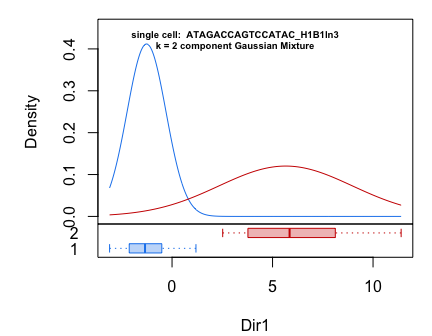Most of the proteins in this fairly large panel are in the background distribution (N1). 14 proteins form the positive staining distribution (N2) in this cell:

table(cell2$classification) #> #> 1 2 #> 73 14 What this means is that the more highly parameterized models (those with a larger number of k values) tend to overfit the background distribution. For example, for this cell. We can compare this k = 2 component mixture to a Gaussian with k = 1 to k= 6 component mixtures. Below the distribution of protein populations inferred to belong to each sub-population is visualized as above for the k = 3 and 6 component mixtures as the Gaussian density distribution that we approximated with the model. models shown below fit to the same cell as above # fit a mixture with between 1 and 6 components. m.list = lapply(as.list(1:6), function(k) Mclust(norm_adt[ ,2], G = k, warn = FALSE, verbose = FALSE )) # extract densities for k = 3 and k = 6 component models dr_3 = MclustDR(m.list[]) dr_6 = MclustDR(m.list[]) # visualize distributiion of protein populations with different k # in Gaussian mixture for a single cell plot.title = paste0('single cell: ', cell.name, '\n k-component Gaussian Mixture ') plot(dr_3,what = "density") title(cex.main = 0.6, plot.title)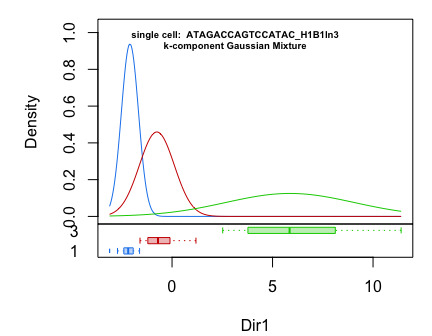plot(dr_6,what = "density") title(cex.main = 0.6, plot.title)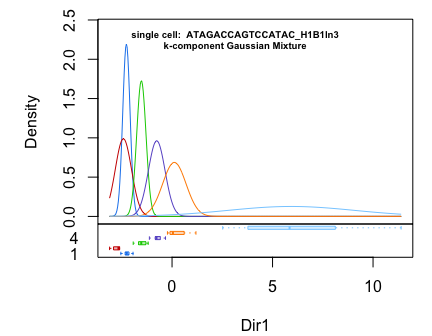The clear positive distribution in all cases is similar and is far from the lower peak; increasing the k value (the number of mixing components) finds many local densities near the background. The variation in background means is low relative to the magnitude of the difference between the background peaks and the clear positive peak. We observed this trend even in datasets with only 14 proteins We can use information criteria to determine the optimal model for the cell. Note that Mclust uses a convention where there is not a - sign in the information criteria calculation for BIC, (which is typically used in a regression context), meaning the model that has the highest relative BIC is the optimal model. We can see that the 2 component mixture has the optimal BIC, and it is very similar to the 3 component model. In the paper we show the distribution of the fitted values for k = 1-6 across cells. We further find that regardless of the optimal model, the µ1 parameter estimated is highly concordant between k = 2 or k = 3 component models. Note all of this is to help justify a single fitted parameter value in the dsb model–this parameter µ1 is further combined with other measures of noise, the isotype control antibodies to form the technical component as outlined below. This makes dsb insensitive to cells which may have true 3 or 4 modal distributions in protein levels because the background mean tends to be very robustly extracted by a 2 component mixture.  plot( sapply(m.list, function(x) x$bic), type = 'b',
ylab = 'Mclust BIC -- higher is better',
xlab = 'mixing components k in Gaussian Mixture model',
main = paste0('single cell:  ', cell.name),
col =r, pch = 18, cex = 1.4, cex.main = 0.8
)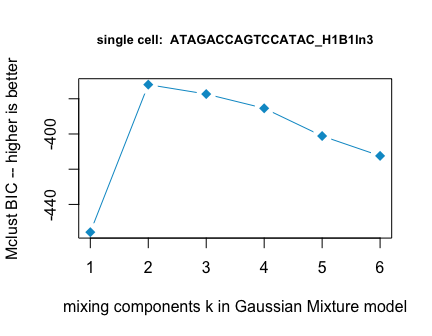## dsb Part II step II

formation of the noise matrix

First dsb extracts the k=2 component mixture µ1 value:


# extract mu 1 as a vector
mu.1 = unlist(
lapply(
cmd, function(x){x$parameters$mean }
)
)

# check distribution of the fitted value for µ1 across cells
hist(mu.1, col = r,breaks = 30)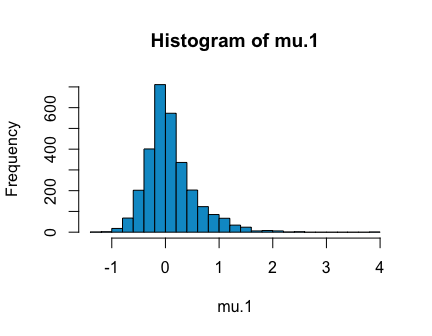As indicated above, we do not use µ1 alone as a correction factor. Instead we utilize the correlation structure between isotype controls and µ1 to derive a technical factor for each cell that anchors the component of the background mean µ1 toward the component associated with technical noise. See the paper for further details.

Create noise matrix for calculating the technical component with µ1 and isotype values.

# define isotype controls
isotype.control.name.vec = c("Mouse IgG2bkIsotype_PROT", "MouseIgG1kappaisotype_PROT",
"MouseIgG2akappaisotype_PROT", "RatIgG2bkIsotype_PROT" )
# construct noise matrix

# transpose to fit PC
tmat = t(noise_matrix)

# view the noise matrix on which to calculate pc1 scores.
#>                                    mu.1 Mouse IgG2bkIsotype_PROT
#> GAACGGATCGAATCCA_H1B1ln2.1  0.424416035                2.0081678
#> ATAGACCAGTCCATAC_H1B1ln3.1  0.129457815                0.1938776
#> GAAGCAGAGTATCTCG_H1B1ln4.1  0.008699038               -0.8412382
#> CTACCCAAGCTACCGC_H1B1ln6.1  0.239007906                0.1938776
#> AGCCTAAAGGATATAC_H1B1ln2.1 -0.170614869                0.1938776
#> CGGACTGGTCCGCTGA_H1B1ln6.1 -0.039058826               -0.8412382
#>                            MouseIgG1kappaisotype_PROT
#> GAACGGATCGAATCCA_H1B1ln2.1                  0.7409978
#> ATAGACCAGTCCATAC_H1B1ln3.1                 -0.6802394
#> GAAGCAGAGTATCTCG_H1B1ln4.1                 -0.6802394
#> CTACCCAAGCTACCGC_H1B1ln6.1                 -0.6802394
#> AGCCTAAAGGATATAC_H1B1ln2.1                 -0.6802394
#> CGGACTGGTCCGCTGA_H1B1ln6.1                  0.7409978
#>                            MouseIgG2akappaisotype_PROT RatIgG2bkIsotype_PROT
#> GAACGGATCGAATCCA_H1B1ln2.1                   2.1388457            -0.4511315
#> ATAGACCAGTCCATAC_H1B1ln3.1                   1.4410413             0.7393731
#> GAAGCAGAGTATCTCG_H1B1ln4.1                  -0.1319484            -0.4511315
#> CTACCCAAGCTACCGC_H1B1ln6.1                  -0.1319484            -0.4511315
#> AGCCTAAAGGATATAC_H1B1ln2.1                   1.4410413             0.7393731
#> CGGACTGGTCCGCTGA_H1B1ln6.1                  -1.0293939            -0.4511315

calculate eigenvector through the noise matrix
Next we calculate PC1 score for cells based on their noise variables. rotation = the loadings, x = the position for each observation along each principal component (the PC scores). These PC scores are the dsb technical component.

# calculate principal component 1
g = prcomp(tmat, scale = TRUE)

# get the dsb technical component for each cell -- PC1 scores (position along PC1) for each cell
head(g$x) #> PC1 PC2 PC3 PC4 #> GAACGGATCGAATCCA_H1B1ln2.1 -2.0901957 2.0749807 -0.64175456 0.05764503 #> ATAGACCAGTCCATAC_H1B1ln3.1 -0.6273177 1.1997987 1.50456704 0.11944273 #> GAAGCAGAGTATCTCG_H1B1ln4.1 1.0445432 0.5002462 0.08893046 -0.05208374 #> CTACCCAAGCTACCGC_H1B1ln6.1 0.2679178 0.4873479 -0.24739624 0.58365532 #> AGCCTAAAGGATATAC_H1B1ln2.1 -0.2224726 1.1748842 1.54873673 0.06409935 #> CGGACTGGTCCGCTGA_H1B1ln6.1 0.8920804 -0.7322072 -0.69982410 -0.78758017 #> PC5 #> GAACGGATCGAATCCA_H1B1ln2.1 -0.9566846 #> ATAGACCAGTCCATAC_H1B1ln3.1 -0.3505210 #> GAAGCAGAGTATCTCG_H1B1ln4.1 0.6115644 #> CTACCCAAGCTACCGC_H1B1ln6.1 0.5164147 #> AGCCTAAAGGATATAC_H1B1ln2.1 -0.8441784 #> CGGACTGGTCCGCTGA_H1B1ln6.1 0.4273887 head(g$rotation)
#>                                    PC1         PC2         PC3          PC4
#> mu.1                        -0.6297845  0.03875738 -0.06871119  0.086093199
#> Mouse IgG2bkIsotype_PROT    -0.4949070 -0.03401375 -0.32125325  0.630195454
#> MouseIgG1kappaisotype_PROT  -0.3896649 -0.31341086 -0.39186390 -0.728176340
#> MouseIgG2akappaisotype_PROT -0.3140363  0.83497701  0.28022795 -0.255256528
#> RatIgG2bkIsotype_PROT       -0.3286045 -0.44936395  0.81239775 -0.006706201
#>                                    PC5
#> mu.1                         0.7679427
#> Mouse IgG2bkIsotype_PROT    -0.5035476
#> MouseIgG1kappaisotype_PROT  -0.2571707
#> MouseIgG2akappaisotype_PROT -0.2459898
#> RatIgG2bkIsotype_PROT       -0.1733667
dsb.technical.component = g$x[ ,1] hist(dsb.technical.component, breaks = 40, col = r)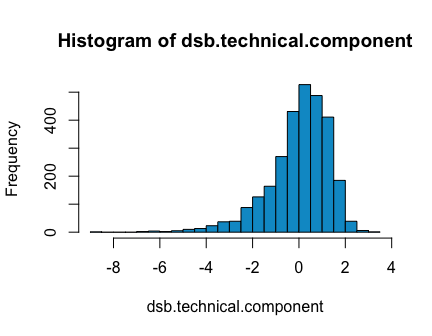Note – the direction of the technical component is arbitrary; only the relative values are important as these are used in a linear model and the residuals are calculated (see below). Fit linear model regressing protein expression on the technical component We now fit a linear model to each cell regressing each protein’s expression on the dsb technical component. dsb uses the residual of this fit plus the intercept for each protein as the dsb normalized values.  # constructs a matrix of 1 by number of columns in norm_adt1 covariate = as.matrix(dsb.technical.component) design = matrix(1, ncol(norm_adt), 1) # fit a linear model to solve the coefficient for each protein with QR decomposition. fit <- limma::lmFit(norm_adt, cbind(design, covariate)) # this subset just extracts the beta for each protein. beta <- fit$coefficients[, -(1:ncol(design)), drop = FALSE]
beta[is.na(beta)] <- 0

# beta is the coefficient for each prot.
plot(beta, col = r , pch = 16, xlab = 'protein')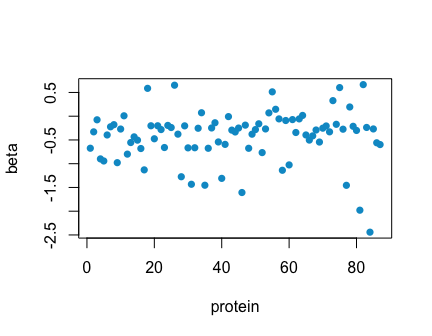calculate residual of linear model fit Finally we ‘regress out’ the technical component. These are the final dsb normalized values.

denoised_adt_2 = as.matrix(norm_adt) - beta %*% t(covariate)

Note, the code above was modified from the limma function removeBatchEffect. Internally dsb uses this function to regress out the technical component for convenience because it is very robust and efficient.

# regress out the technical component using limma
# note this is how limma (and dsb) calculates this
denoised_adt = limma::removeBatchEffect(norm_adt, covariates = dsb.technical.component)

We can confirm these steps above are equivalent to what is output from the dsb function.

# default dsb call
empty_drop_matrix = neg,
denoise.counts = TRUE,
isotype.control.name.vec = isotype.control.name.vec)
#>  "correcting ambient protein background noise"
#>  "some proteins with low background variance detected check raw and normalized distributions.  protein stats can be returned with return.stats = TRUE"
#>  "CD185_PROT"
#>  "fitting models to each cell for dsb technical component and removing cell to cell technical noise"

You can confirm that these are all the same all.equal(denoised_adt, denoised_adt_2, denoised_adt_3)

### How can I interpret the dsb values?

Because of the transformation in step 1 , the scale of the values can be interpreted similar to signal to ambient noise ratios. With step 1 + 2, we can interpret the values as signal to noise ratios with technical, non-biological cell-to cell variations removed. This means we can set principled cutoffs, rather than just drawing arbitrary lines as what is positive and negative, we can say with confidence any cells with a protein level below, 3 or 3.5 (you can pick based on the distribution of values in your dataset) are negative for the protein.

These dsb normalized values can be used directly (for example, without further standardizing) protein based or joint protein and RNA clustering (see main vignette). We have also found these values are highly interpretable for use in machine learning and other regression approaches for modeling protein expression in single cell data measuring ADTs.


qplot(as.data.frame(t(denoised_adt))$CD3_PROT, as.data.frame(t(denoised_adt))$CD19_PROT) +
plist +
ggtitle('dsb step 1 + 2 normalized and denoised') +
geom_vline(xintercept = 3.5, color = 'red') +
geom_hline(yintercept = 3.5, color = 'red')